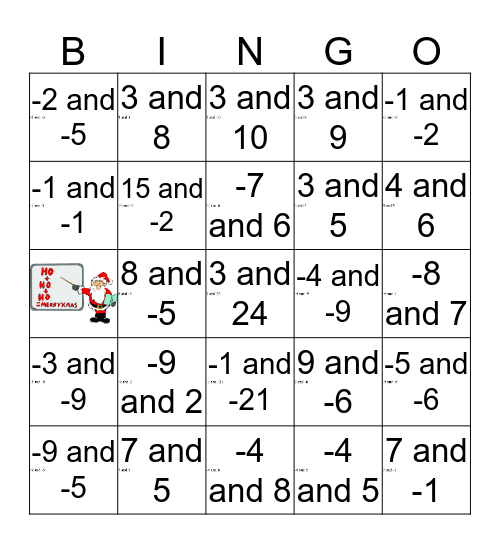# That's Sum Product 3This bingo card has 25 images and 24 words: -9 and 2, -4 and 8, -7 and 6, -9 and -5, -1 and -2, -2 and -5, -1 and -1, 3 and 8, -4 and -9, -1 and -21, 3 and 5, 9 and -6, -5 and -6, 15 and -2, 7 and -1, -8 and 7, 3 and 10, 4 and 6, 8 and -5, 3 and 24, 7 and 5, -3 and -9, 3 and 9 and -4 and 5.

## Play Online